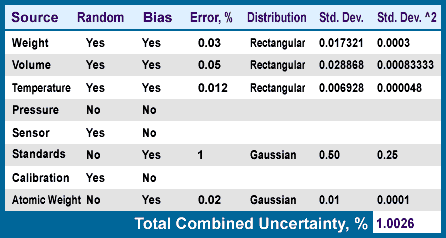Cart

# Understanding Error Budgets

The topic of Error Budgets is one that should be of concern to anyone using or manufacturing certified reference materials (CRMs). This article explains the use of an Error Budget regarding the accounting of all significant errors in our production of CRMs and the calculation of certified value(s) uncertainties.

## Defining the Terms

The term Error Budget is used in this article to be a listing of every possible source of random and fixed errors with identification as to their classification, absolute values, and distribution (rectangular or Gaussian). The following list covers additional terms that are commonly encountered when working with Error Budgets:

• Accuracy is the nearness of a result (Xi) or the arithmetic mean (M) of a set of results to the true value (µ).
• Error is the term used to express accuracy, Xi - µ (or M - µ). It is the difference between the true value (unknown) and the experimental value or mean.
• Absolute Error is equal to Xi - µ and is expressed in the dimension of the two numbers. The absolute error for weighing on one of our four-place analytical balances is 0.0001 grams up to 200 grams.
• Relative Error is the absolute error divided by the true value (µ). The relative error is calculated from the absolute error and the actual measurement (weight in the above example).
• Systematic Errors are always of the same sign and magnitude and produce bias. They are constant no matter how many measurements are made. We commonly refer to them as Fixed Errors.
• Random Errors vary in sign and magnitude and are unpredictable. They occur by chance. Random errors average out and approach zero if enough measurements are made.
• Blunders are simply mistakes that occur on occasion and produce erroneous results that are outliers and may be recognized as such by statistical procedures. They cannot be treated by statistics.
• Precision is the agreement of a set of results among themselves. Precision is expressed in terms of the deviation of a set of experimental results from the arithmetic mean of the set.
• Deviation is the difference between the mean and an individual result.
• Average Deviation refers to the summation of the deviation divided by the number of results.
• Standard Deviation of a sample is the square root of the average of the square of the individual deviations from the mean divided by n-1, where n is the number of measurements.
• Uncertainty is an estimate of error in mathematical terms. This expression must be defined exactly and is typically expressed using the standard deviation. Where error is the difference between the true value and a result or the mean, the uncertainty is an expression involving a calculation where the deviation (difference between mean and individual result) is used. The hope is that the true value (which will never be known by man) is within the expressed uncertainty under the specified conditions.

## Sources of Uncertainty

In the following example, the standard deviations are calculated from the % Relative Errors for each measurement. We have identified the sources of error for each of our test methods and have incorporated the calculations for the uncertainty of each measurement into a spreadsheet that has been written for each test method type, i.e., there are many gravimetric procedures, but only one spreadsheet is needed.

In figure 1 below, the Error, % is termed in our procedures as the Relative Error and is not converted to % units. For example, if you weigh a 0.1 gram weight on a four-place balance, the Relative Error is 0.0001 / 0.1 = 0.001. However the Error, % is 0.1 (i.e. - the Relative Error multiplied by 100).

Figure 1 - Calculations and Results:The Relative Errors are calculated by the analyst and entered in the database worksheet. The worksheet then multiplies the Relative Error by the Mean to get the error in absolute terms such as µg/mL. The Random Error of measurement is then added to this error using the square root of the sum of the squares of all the Relative Errors identified in the worksheet. The final uncertainty is calculated by multiplying this value by 2 and dividing by the square root of the number of measurements.

## Further Information

To learn more about Error Budgets and their use in within our industry, contact us and we'll be happy to recommend additional resources.